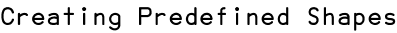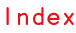RSS

Scriptographer allows you to create path items with predefined shapes of variable dimensions.

In the same way that the new Path() constructor creates an empty path with no points, we have constructors such as new Path.Circle(center, radius) and new Path.Rectangle(point, size) that create paths and automatically add segments to them to create predefined shapes.

## Circle Shaped Paths

Using the new Path.Circle(center, radius) constructor, we can produces circle shaped paths.

The following code produces a circle shaped path with a center point at {x: 100, y: 100} and a radius of 50 pt:

```var myCircle = new Path.Circle(new Point(100, 100), 50);
```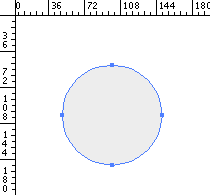## Rectangular Shaped Paths

To create a rectangular shaped path you can pass a Rectangle to the new Path.Rectangle(rect) constructor.

A Rectangle object is an abstract representation of a rectangle. Please read the Point, Size and Rectangle tutorial to find out how to work with the Rectangle object.

For example, let's make a Rectangle between {x: 0, y: 0} and {x: 50, y: 50} and then use the new Path.Rectangle(rect) constructor to create a path with the shape that it describes:

```var rectangle = new Rectangle(new Point(50, 50), new Point(150, 100));
var path = new Path.Rectangle(rectangle);
```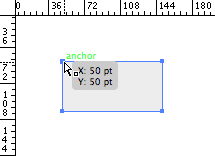## Rectangular Shaped Paths with Rounded Corners

To create rectangular shaped paths with rounded corners, we use the new Path.RoundedRectangle(rect, size) constructor. The rect parameter describes the Rectangle and the size parameter describes the Size of the rounded corners.

For example, the following script creates a rectangular shaped path with 10 pt * 10 pt corners:

```var rectangle = new Rectangle(new Point(50, 50), new Point(150, 100));
var cornerSize = new Size(10, 10);
var path = new Path.RoundRectangle(rectangle, cornerSize);
```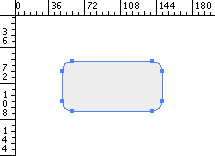## Regular Polygon Shaped Paths

To create regular polygon shaped paths, we use the new Path.RegularPolygon(center, numSides, radius) constructor.

The center parameter describes the center point of the polygon, the numSides parameter describes the amount of sides the polygon has and the radius parameter describes the radius of the polygon.

For example, lets create a triangle shaped path and a decahedron shaped path:

```// Create a triangle shaped path
var triangle = new Path.RegularPolygon(new Point(80, 100), 3, 50);

// Create a decahedron shaped path
var decahedron = new Path.RegularPolygon(new Point(200, 100), 10, 50);
```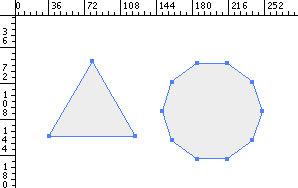Did you know?

You can check the constructors section of the Path reference for a full list of Path constructors.